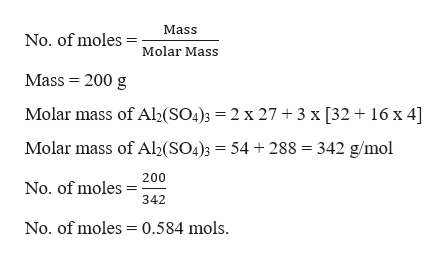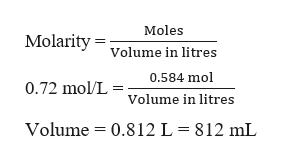# Calculate the volume in milliliters of a 0.72/molL aluminum sulfate solution that contains 200.g of aluminum sulfate (Al2(SO4)3)Be sure your answer has the correct number of significant digits.

Question
22 views

Calculate the volume in milliliters of a 0.72/molL aluminum sulfate solution that contains 200.g of aluminum sulfate (Al2(SO4)3)

check_circle

Step 1

It is required to calculate the volume of 0.72 mol/L aluminium sulfate solution containing 200 g of aluminium sulfate. This can be done as,help_outlineImage TranscriptioncloseMass No. of moles Molar Mass Mass 200 g Molar mass of Al2(SO4)3 = 2 x 27 + 3 x [32 16 x 4] Molar mass of Al2(SO4)3 = 54 + 288 342 g/mol 200 No. of moles 342 No. of moles 0.584 mols fullscreen
Step 2

The volume can be ca...help_outlineImage TranscriptioncloseMoles Molarity Volume in litres 0.584 mol 0.72 mol/L = Volume in litres Volume 0.812 L = 812 mL fullscreen

### Want to see the full answer?

See Solution

#### Want to see this answer and more?

Solutions are written by subject experts who are available 24/7. Questions are typically answered within 1 hour.*

See Solution
*Response times may vary by subject and question.
Tagged in

### General Chemistry